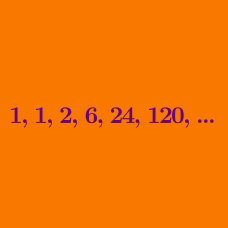Number Theory

# Wilson's Theorem

What is $$28 ! \pmod{29} ?$$

What is $$59 ! \pmod{61} ?$$

What is $$(18 !)^2 \pmod{19} ?$$

What is $$(10 !)^ {84} \pmod{11} ?$$

If $$(n-1)! \equiv -1 \pmod{n} ,$$ where $$n$$ is an integer larger than one, which of the following is true?

×